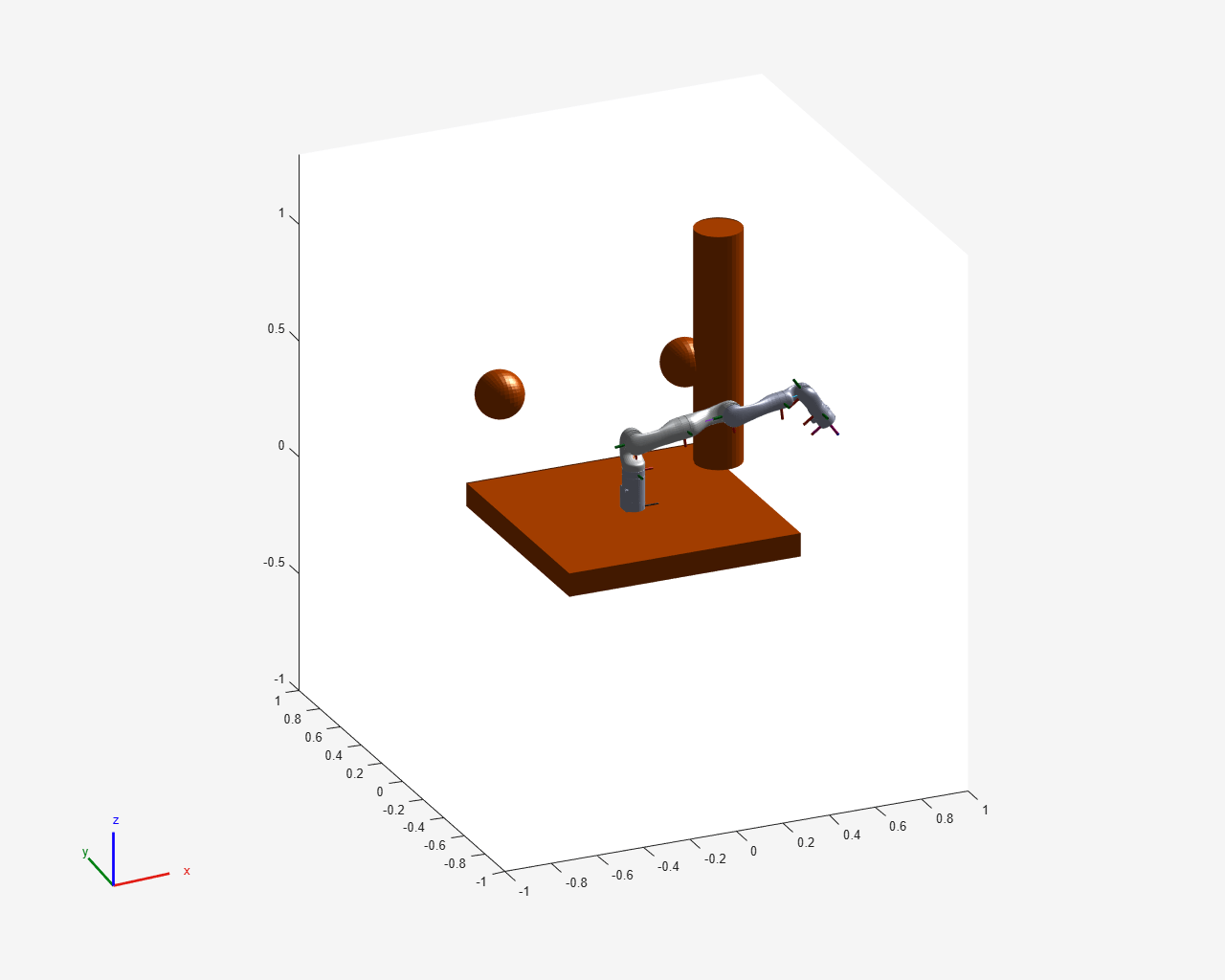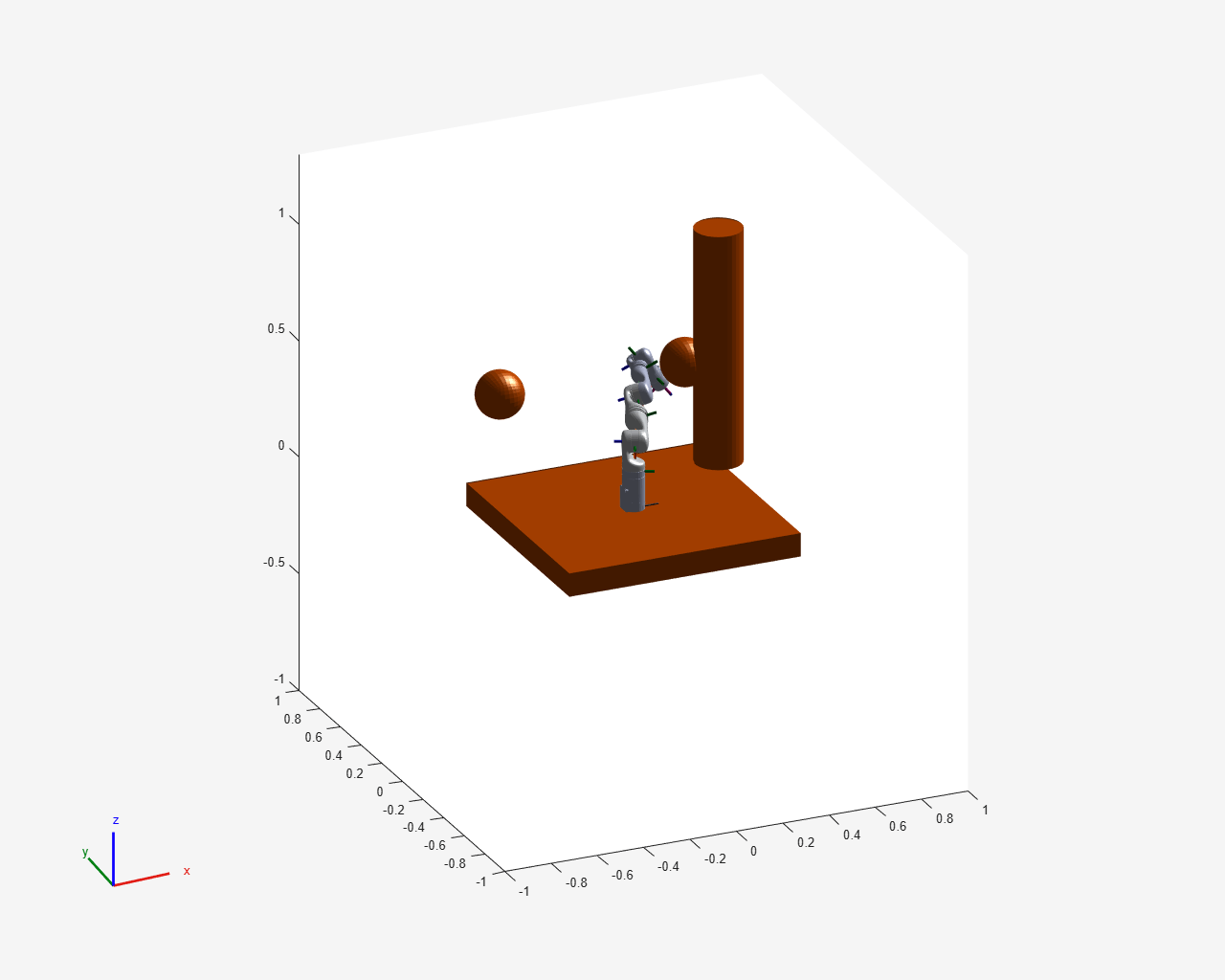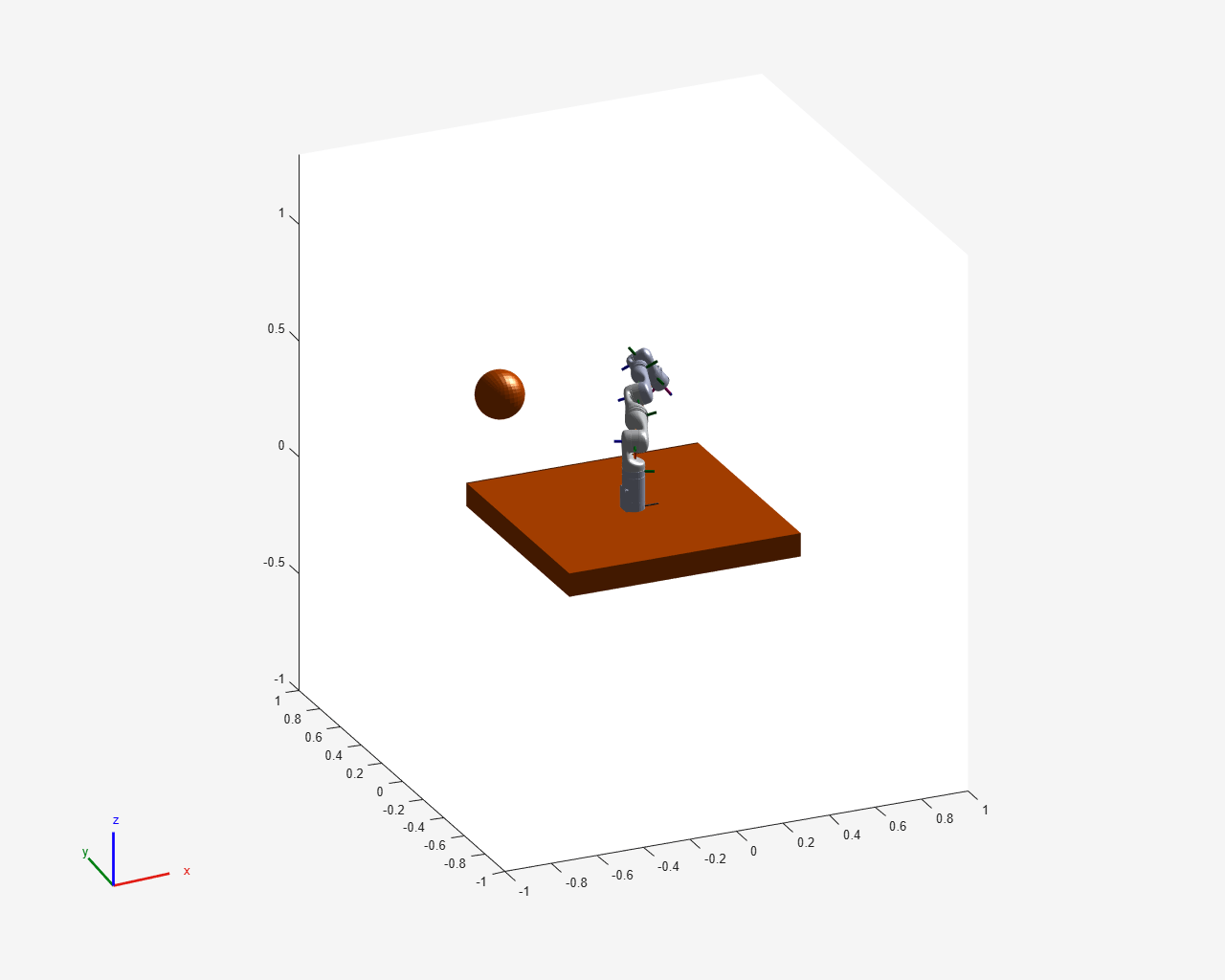# Generate Code for Manipulator Motion Planning in Perceived Environment

This example shows how to generate code for planning manipulator motion in a perceived environment. Perceived environments can have a variable number of collision objects that can be a combination of heterogeneous types (spheres, cylinders, meshes, and boxes). This example uses the `convertToCollisionMesh` function to homogenize the cell array of collision objects by converting the primitive type objects to their mesh equivalents.

### Set Up Robot and Environment

In this example, you generate a MEX function for a MATLAB function that uses a `manipulatorRRT` object to plan for a Kinova Gen 3 robot.

Create a rigid body tree object to model the robot. For this example, load a Kinova Gen3 manipulator.

`kinova = loadrobot("kinovaGen3",DataFormat="row");`

#### Create Environment

Real perception subsystems can output a structure that specifies the type of perceived geometry, its dimensions, and the pose of the object in the base frame of the robot. To simulate this, first create a structure to contain collision objects.

```geomStructType = struct("Type",exampleHelperCollisionEnum.Box, ... "X",0, ... "Y",0, ... "Z",0, ... "Vertices",coder.typeof(zeros(3),[inf 3],[1 0]), ... "Radius",0, ... "Height",0, ... "Pose",eye(4)); geomStruct = geomStructType; geomStruct.Vertices=zeros(3);```

Next create a cylinder, a box, and two spheres, as perceived by a perception subsystem.

Use `geomStruct` as the framework to create a perceived box with `X`, `Y`, and `Z` lengths of `1`, `1`, and `0.1`, respectively.

```boxGeom = geomStruct; boxGeom.Type = exampleHelperCollisionEnum.Box; boxGeom.X = 1; boxGeom.Y = 1; boxGeom.Z = 0.1; boxGeom.Pose = trvec2tform([0 0 -0.051]);```

Create a perceived cylinder of radius `1` and height `0.1`.

```cylinderGeom = geomStruct; cylinderGeom.Type = exampleHelperCollisionEnum.Cylinder; cylinderGeom.Radius = 0.1; cylinderGeom.Height = 1.0; cylinderGeom.Pose = trvec2tform([0.5 0.3 0.50]);```

Create two perceived spheres, each with a radius of `0.1`.

```sphere1Geom = geomStruct; sphere1Geom.Type = exampleHelperCollisionEnum.Sphere; sphere1Geom.Radius = 0.1; sphere1Geom.Pose = trvec2tform([0.4 0.4 0.4]); sphere2Geom = geomStruct; sphere2Geom.Type = exampleHelperCollisionEnum.Sphere; sphere2Geom.Radius = 0.1; sphere2Geom.Pose = trvec2tform([-0.4 0.4 0.4]);```

### Set Up Planning Variables

The, `exampleHelperVariableHeterogeneousPlanner` helper function accepts the start and goal joint configurations of the robot, as well as an array of structure elements representing the environment. In this example, the start and goal joint configurations are set arbitrarily, but you can set them as necessary.

```startConfig = [-0.0035 1.4453 0.0334 0.0144 -0.0294 1.0600 -0.0077]; goalConfig = [-1.1519 1.5243 0.3186 0.0009 0.2466 1.2090 -1.1952]; env1 = [boxGeom cylinderGeom sphere1Geom sphere2Geom];```

Visualize the start configuration of the robot in the perceived environment `env1`.

```figure(Name="Env1",Visible="on",Units="normalized",OuterPosition=[0 0 1 1]) exampleHelperVisualizeVarSizeEnvironment(env1,kinova,startConfig);```### Generate Code for Planning

In order to generate a MEX function, `exampleHelperVariableHeterogeneousPlanner_mex`, from the MATLAB function `exampleHelperVariableHeterogeneousPlanner`, run this command:

```codegen("exampleHelperVariableHeterogeneousPlanner", ... "-args",{startConfig,goalConfig,coder.typeof(geomStruct,[1 inf],[0 1])}) ```

The `variable_dims` argument of `coder.typeof` (Fixed-Point Designer) specifies that the input row vector `geomStruct` of the entry-point planning function can have an unbounded and variable-sized second dimension.

### Plan Collision-Free Path

Next, use the planner entry-point helper function to output a geometric collision-free plan.

`planInEnv1 = exampleHelperVariableHeterogeneousPlanner(startConfig,goalConfig,env1);`

You can also reuse the `exampleHelperVariableHeterogeneousPlanner` for a different perceived environment. Plan in a new environment, `env2`, created by removing `cylinderGeom` and `sphereGeom` from `env1. `

```env2 = [boxGeom,sphere2Geom]; planInEnv2 = exampleHelperVariableHeterogeneousPlanner(startConfig,goalConfig,env2);```

MEX function for planning in `env2`, and do not need to generate it again. It has the same behavior `exampleHelperVariableHeterogeneousPlanner`, but results in shorter planning times.

To use the MEX function instead, run this code:

```planInEnv1 = exampleHelperVariableHeterogeneousPlanner_mex(startConfig,goalConfig,env1); env2 = [boxGeom sphere2Geom]; planInEnv2 = exampleHelperVariableHeterogeneousPlanner_mex(startConfig,goalConfig,env2); ```

### Visualize Planned Path

Visualize the planned output in both `env1` and `env2.`

```figure(Name="Planned path in env1",Visible="on",Units="normalized",OuterPosition=[0,0,1,1]) exampleHelperVisualizeVarSizeEnvironment(env1,kinova,planInEnv1);``````figure(Name="Planned path in env2",Visible="on",Units="normalized",OuterPosition=[0,0,1,1]) exampleHelperVisualizeVarSizeEnvironment(env2,kinova,planInEnv2);```### Supporting Functions

#### Planner Entry Point Function

`exampleHelperVariableHeterogeneousPlanner` plans motion in the perceived environment of a Kinova Gen 3 robot workspace. The helper function accepts the perceived environment as the `geomStructs` argument, which is an array of structures where each element contains the information used to represent a collision object. Make the rigid body tree of the robot persistent. This improves the speed of the mexed function because you only need to create the robot once when you use the function multiple times.

The input structure array `geomStructs` is variable in size and consists of structure elements that each represent the collision geometry defined by the `Type` field of the structure. The `Type` field stores an enumeration, `exampleHelperCollisionEnum`, specifying whether the perceived object is a `Box`, `Sphere`, `Cylinder` or `Mesh`. For more information on how to construct a collision object structure, see Create Environment.

The second `manipulatorRRT` input argument can hold up to 100 objects. Upper bounding is necessary to enable construction of collision objects `collisionBox`, `collisionCylinder`, `collisionSphere`, `collisionMesh` inside a for loop.

```function interpolatedPlan = exampleHelperVariableHeterogeneousPlanner(start,goal,geomStructs) %This function is for internal use only and may be removed in the future. %exampleHelperVariableHeterogeneousPlanner Plan in a perceived environment of a Kinova Gen 3 robot % INTEPROLATEDPLAN=exampleHelperVariableHeterogeneousPlanner(START,GOAL,GEOMSTRUCTS) % Outputs a collision free geometric plan, INTEPROLATEDPLAN, of a Kinova % Gen3 robot in an environment defined by GEOMSTRUCTS which is a variable % sized array of struct elements that capture the information of a % collision geometry in the environment. Each struct element in % GEOMSTRUCTS is of the form: % geomStruct=struct("Type",exampleHelperCollisionEnum.Box,... % "X",0, ... % "Y",0, ... % "Z",0, ... % "Vertices",zeros(3),... % "Radius",0, ... % "Height",0, ... % "Pose",eye(4)); % START and GOAL are the start and goal joint configurations of the % robot, respectively, and are specified as a row vector. %Copyright 2021-2023 The MathWorks, Inc. % Create a placeholder variable for environment which is a cell-array % of collision meshes with vertices that are variably sized. The size % of the environment is that of the input array of collision geometry % struct elements. coder.varsize("vertices",[inf 3],[1 0]); vertices = zeros(3); env = repmat({collisionMesh(vertices)},1,length(geomStructs)); % Load the rigid body tree for which the planner will be defined and make it persistent persistent rbt if isempty(rbt) rbt = loadrobot("kinovaGen3",DataFormat="row"); end % Set up the environment. The maximum number of collision objects that % the environment can hold is 100. for i = coder.unroll(1:100) if (i <= length(geomStructs)) % For each struct element, create the corresponding collision % object (collisonBox, collisionCylinder, collisionSphere, or % collisionMesh) and convert that to its corresponding mesh % equivalent thereby homogenizing the environment. s = geomStructs(i); if s.Type == exampleHelperCollisionEnum.Box env{i} = convertToCollisionMesh( ... collisionBox(s.X,s.Y,s.Z)); elseif s.Type == exampleHelperCollisionEnum.Sphere env{i} = convertToCollisionMesh( ... collisionSphere(s.Radius)); elseif s.Type == exampleHelperCollisionEnum.Cylinder env{i} = convertToCollisionMesh( ... collisionCylinder(s.Radius,s.Height)); elseif s.Type == exampleHelperCollisionEnum.Mesh env{i} = collisionMesh(s.Vertices); end % Assign the pose of the element. env{i}.Pose = s.Pose; end end % Create and set up the planner from the rigid body tree and % environment. planner = manipulatorRRT(rbt,env); planner.SkippedSelfCollisions = "parent"; planner.MaxConnectionDistance = 0.4; planner.ValidationDistance = 0.05; % For repeatable results, seed the random number generator and store % the current seed value. prevseed = rng(0); % Plan and interpolate. planOut = planner.plan(start,goal); interpolatedPlan = planner.interpolate(planOut); % Restore the random number generator to the previously stored seed. rng(prevseed); end ```